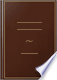بحث صور خرائط YouTube الأخبار Gmail Drive تقويم المزيد »
تسجيل الدخول
 الكتب الكتب 21 - 30 من 192 فيIf a straight line be divided into any two parts, the square of the whole line is....If a straight line be divided into any two parts, the square of the whole line is equal to the squares of the two parts, together with twice the rectangle contained by the parts.The Elements of Euclid: Viz. the First Six Books, Together with the Eleventh ... - الصفحة 41
بواسطة Euclides - 1816 - عدد الصفحات: 528
عرض كامل - حول هذا الكتاب## The Elements of Euclid: Viz. the First Six Books, Together with the Eleventh ...

Euclid, Robert Simson - 1821 - عدد الصفحات: 516
...BC. If therefore a straight line, &c. QED PROP. IV. THEOR. IP a straight line be divided into any twq parts, the square of the whole line is equal to the squares of the two pai ts, together with twice the rectangle contained by the parts. Let the straight line AB be divided...
عرض كامل - حول هذا الكتاب## The Youth's instructer [sic] and guardian

1854
...product. Geometry furnishes us with a parallel to both these cases ; for, as Euclid tells us (II., 4), if a straight line be divided into any two parts, the...together with twice the rectangle contained by the parts ; and, were we to represent the line as a divided into two parts m, x, the algebraical expression for...
عرض كامل - حول هذا الكتاب## A Course of Mathematics: For the Use of Academies, as Well as Private ...

Charles Hutton - 1822
...up of the four figures, viz. the two squares AF, FD, and the two equal rectangles EF, FB. That is, the square of AB is equal to the squares of AC, CB, together with twice the rectangle of AC, CB. ft. ED Corol. Hence, if a line be divided into two equal...
عرض كامل - حول هذا الكتاب## Elements of algebra, compiled from Garnier's French translation of L. Euler ...

Charles Tayler - 1824
...iv. Book ii., viz., that If a number be divided into any two parts, the square of the •whole number is equal to the squares of the two parts, together with twice the product (or rectangle) of the twojiarts. For (c) Prime numbers are such as cannot be represented by...
عرض كامل - حول هذا الكتاب## A Popular Course of Pure and Mixed Mathematics ...: With Tables of ...

Peter Nicholson - 1825 - عدد الصفحات: 372
...', If a straight line be divided into any two parts, the square or in* ELEMENTS OP KUCUD. 191 vhole line is equal to the squares of the two parts, together...straight line AB be divided into any two parts in С ; the square of AB is equnl to the squares of AC, CB, and to twice the rectangle contained by AC,...
عرض كامل - حول هذا الكتاب## Elements of Geometry: Containing the First Six Books of Euclid, with a ...

Euclid, John Playfair - 1826 - عدد الصفحات: 320
...therefore AB.BC = AC.CB -f BC3. Therefore, if a straight line, &e. QED C B D E C PROP. IV. THEOR. If a straight line be divided into any two parts, the...equal to the squares of the two parts, together with twiee the reetangle eontained by the parts. Let the straight line AB be divided into any two parts...
عرض كامل - حول هذا الكتاب## Elements of Geometry, Containing the First Six Books of Euclid

Euclid, Phillips - 1826 - عدد الصفحات: 180
...and it be ag =fg + g2. Q- ED PROPOSITION IV. THEOREM. If a right line be divided into any two part:!, the square of the whole line is equal to the squares...parts, together with twice the rectangle contained under the parts. Let the right line AB be divided into any two parts in the point c ; the square of...
عرض كامل - حول هذا الكتاب## An Introduction to Algebra: Being the First Part of a Course of Mathematics ...

Jeremiah Day - 1827 - عدد الصفحات: 332
...than in ordinary language. The proposition, (Euc. 4- 2.) that when a straight line is divided into two parts, the Square of the whole line is equal to...squares of the two parts, together with twice the product of the parts, is demonstrated, by involving a binomial. Let the side of a square be represented...
عرض كامل - حول هذا الكتاب## The Elements of Euclid: The Errors by which Theon, Or Others, Have Long ...

Robert Simson - 1827 - عدد الصفحات: 513
...twice the rectangle contained by the whole and that part, together with the square of the other part. Let the straight line AB be divided into any two parts in the point C: the squares of AB, BC shall be equal to twice the rectangle AB, BC, together with the...
عرض كامل - حول هذا الكتاب## The First Six Books of the Elements of Euclid, with a Commentary and ...

Euclid, Dionysius Lardner - 1828 - عدد الصفحات: 324
...rectangle under either of them and a third of the other, #c. #c. PROPOSITION II. THEOREM. (223) If a right line (AB) be divided into any two parts (in C), the square of the whole line is equal to the sum of the rectangles under the whole (AB) and each of the parts (AC,...
عرض كامل - حول هذا الكتاب